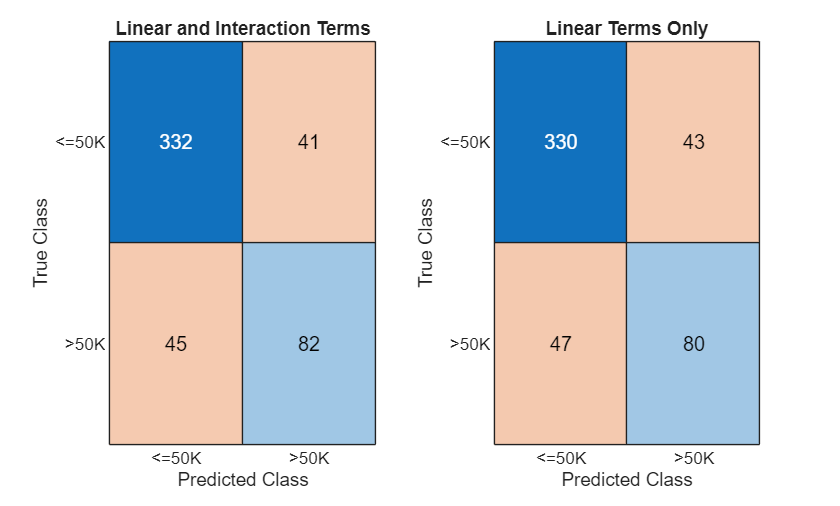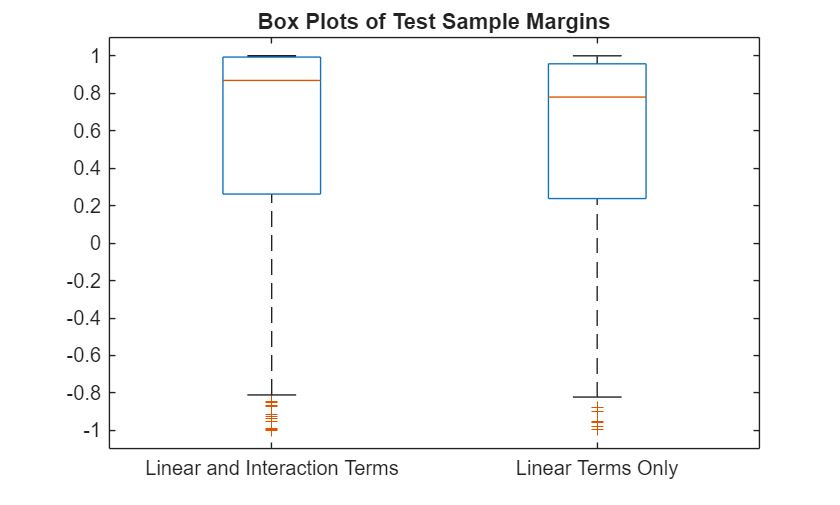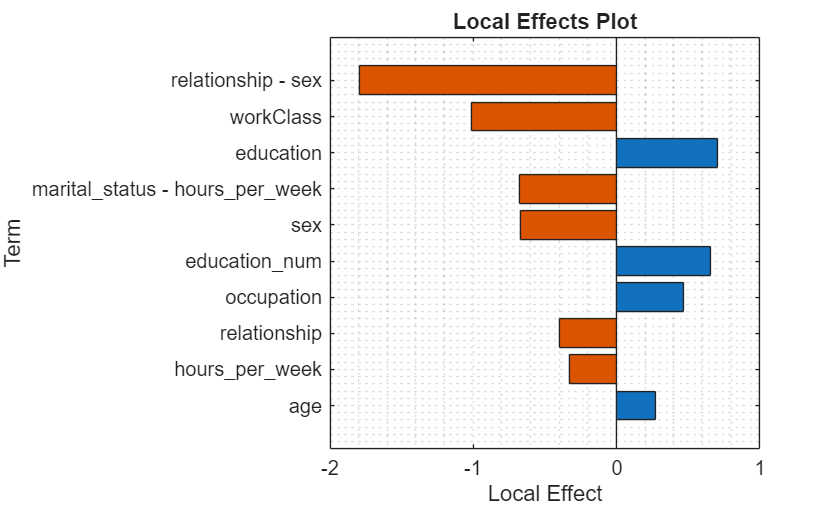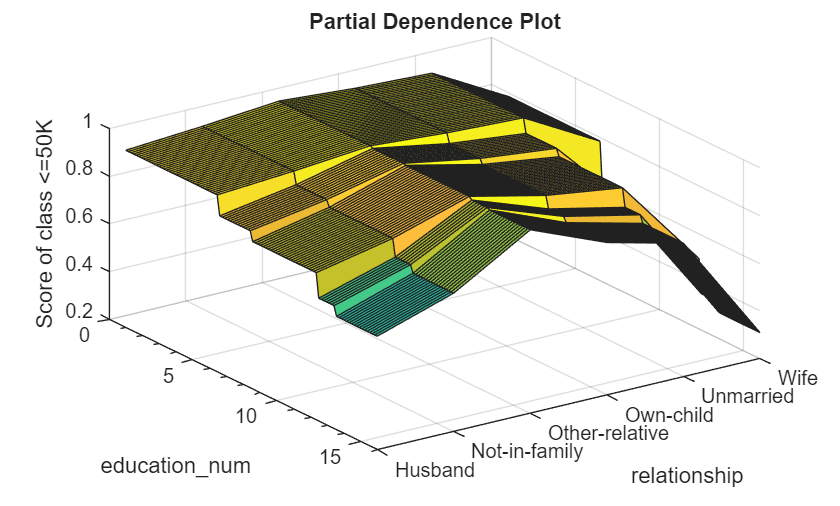# Train Generalized Additive Model for Binary Classification

This example shows how to train a Generalized Additive Model (GAM) for Binary Classification with optimal parameters and how to assess the predictive performance of the trained model. The example first finds the optimal parameter values for a univariate GAM (parameters for linear terms) and then finds the values for a bivariate GAM (parameters for interaction terms). Also, the example explains how to interpret the trained model by examining local effects of terms on a specific prediction and by computing the partial dependence of the predictions on predictors.

Load the 1994 census data stored in `census1994.mat`. The data set consists of demographic data from the US Census Bureau to predict whether an individual makes over \$50,000 per year. The classification task is to fit a model that predicts the salary category of people given their age, working class, education level, marital status, race, and so on.

`load census1994`

`census1994` contains the training data set `adultdata` and the test data set `adulttest`. To reduce the running time for this example, subsample 500 training observations and 500 test observations by using the `datasample` function.

```rng(1) % For reproducibility NumSamples = 5e2; adultdata = datasample(adultdata,NumSamples,'Replace',false); adulttest = datasample(adulttest,NumSamples,'Replace',false);```

### Train GAM with Optimal Hyperparameters

Train a GAM with hyperparameters that minimize the cross-validation loss by using the OptimizeHyperparameters name-value argument.

You can specify `OptimizeHyperparameters` as `'auto'` or `'all'` to find optimal hyperparameter values for both univariate and bivariate parameters. Alternatively, you can find optimal values for univariate parameters using the `'auto-univariate'` or `'all-univariate'` option, and then find optimal values for bivariate parameters using the `'auto-bivariate'` or `'all-bivariate'` option. This example uses `'auto-univariate'` and `'auto-bivariate'`.

Train a univariate GAM. Specify `OptimizeHyperparameters` as `'auto-univariate'` so that `fitcgam` finds optimal values of the `InitialLearnRateForPredictors` and `NumTreesPerPredictor` name-value arguments. For reproducibility, use the `'expected-improvement-plus'` acquisition function. Specify `ShowPlots` as `false` and `Verbose` as 0 to disable plot and message displays, respectively.

```Mdl_univariate = fitcgam(adultdata,'salary','Weights','fnlwgt', ... 'OptimizeHyperparameters','auto-univariate', ... 'HyperparameterOptimizationOptions',struct('AcquisitionFunctionName','expected-improvement-plus', ... 'ShowPlots',false,'Verbose',0))```
```Mdl_univariate = ClassificationGAM PredictorNames: {'age' 'workClass' 'education' 'education_num' 'marital_status' 'occupation' 'relationship' 'race' 'sex' 'capital_gain' 'capital_loss' 'hours_per_week' 'native_country'} ResponseName: 'salary' CategoricalPredictors: [2 3 5 6 7 8 9 13] ClassNames: [<=50K >50K] ScoreTransform: 'logit' Intercept: -1.3118 NumObservations: 500 HyperparameterOptimizationResults: [1×1 BayesianOptimization] Properties, Methods ```

`fitcgam` returns a `ClassificationGAM` model object that uses the best estimated feasible point. The best estimated feasible point indicates the set of hyperparameters that minimizes the upper confidence bound of the objective function value based on the underlying objective function model of the Bayesian optimization process. You can obtain the best point from the `HyperparameterOptimizationResults` property or by using the `bestPoint` function.

`x = Mdl_univariate.HyperparameterOptimizationResults.XAtMinEstimatedObjective`
```x=1×2 table InitialLearnRateForPredictors NumTreesPerPredictor _____________________________ ____________________ 0.02257 118 ```
`bestPoint(Mdl_univariate.HyperparameterOptimizationResults)`
```ans=1×2 table InitialLearnRateForPredictors NumTreesPerPredictor _____________________________ ____________________ 0.02257 118 ```

For more details on the optimization process, see Optimize GAM Using OptimizeHyperparameters.

Train a bivariate GAM. Specify `OptimizeHyperparameters` as `'auto-bivariate'` so that `fitcgam` finds optimal values of the `Interactions`, `InitialLearnRateForInteractions`, and `NumTreesPerInteraction` name-value arguments. Use the univariate parameter values in `x` so that the software finds optimal parameter values for interaction terms based on the x values.

```Mdl = fitcgam(adultdata,'salary','Weights','fnlwgt', ... 'InitialLearnRateForPredictors',x.InitialLearnRateForPredictors, ... 'NumTreesPerPredictor',x.NumTreesPerPredictor, ... 'OptimizeHyperparameters','auto-bivariate', ... 'HyperparameterOptimizationOptions',struct('AcquisitionFunctionName','expected-improvement-plus', ... 'ShowPlots',false,'Verbose',0))```
```Mdl = ClassificationGAM PredictorNames: {'age' 'workClass' 'education' 'education_num' 'marital_status' 'occupation' 'relationship' 'race' 'sex' 'capital_gain' 'capital_loss' 'hours_per_week' 'native_country'} ResponseName: 'salary' CategoricalPredictors: [2 3 5 6 7 8 9 13] ClassNames: [<=50K >50K] ScoreTransform: 'logit' Intercept: -1.4587 Interactions: [6×2 double] NumObservations: 500 HyperparameterOptimizationResults: [1×1 BayesianOptimization] Properties, Methods ```

Display the optimal bivariate hyperparameters.

`Mdl.HyperparameterOptimizationResults.XAtMinEstimatedObjective`
```ans=1×3 table Interactions InitialLearnRateForInteractions NumTreesPerInteraction ____________ _______________________________ ______________________ 6 0.0061954 422 ```

The model display of `Mdl` shows a partial list of the model properties. To view the full list of the model properties, double-click the variable name `Mdl` in the Workspace. The Variables editor opens for `Mdl`. Alternatively, you can display the properties in the Command Window by using dot notation. For example, display the `ReasonForTermination` property.

`Mdl.ReasonForTermination`
```ans = struct with fields: PredictorTrees: 'Terminated after training the requested number of trees.' InteractionTrees: 'Terminated after training the requested number of trees.' ```

You can use the `ReasonForTermination` property to determine whether the trained model contains the specified number of trees for each linear term and each interaction term.

Display the interaction terms in `Mdl`.

`Mdl.Interactions`
```ans = 6×2 5 12 1 6 6 12 1 12 7 9 2 6 ```

Each row of `Interactions` represents one interaction term and contains the column indexes of the predictor variables for the interaction term. You can use the `Interactions` property to check the interaction terms in the model and the order in which `fitcgam` adds them to the model.

Display the interaction terms in `Mdl` using the predictor names.

`Mdl.PredictorNames(Mdl.Interactions)`
```ans = 6×2 cell {'marital_status'} {'hours_per_week'} {'age' } {'occupation' } {'occupation' } {'hours_per_week'} {'age' } {'hours_per_week'} {'relationship' } {'sex' } {'workClass' } {'occupation' } ```

### Assess Predictive Performance on New Observations

Assess the performance of the trained model by using the test sample `adulttest` and the object functions `predict`, `loss`, `edge`, and `margin`. You can use a full or compact model with these functions.

If you want to assess the performance of the training data set, use the resubstitution object functions: `resubPredict`, `resubLoss`, `resubMargin`, and `resubEdge`. To use these functions, you must use the full model that contains the training data.

Create a compact model to reduce the size of the trained model.

```CMdl = compact(Mdl); whos('Mdl','CMdl')```
``` Name Size Bytes Class Attributes CMdl 1x1 5126918 classreg.learning.classif.CompactClassificationGAM Mdl 1x1 5272831 ClassificationGAM ```

Predict labels and scores for the test data set (`adulttest`), and compute model statistics (loss, margin, and edge) using the test data set.

```[labels,scores] = predict(CMdl,adulttest); L = loss(CMdl,adulttest,'Weights',adulttest.fnlwgt); M = margin(CMdl,adulttest); E = edge(CMdl,adulttest,'Weights',adulttest.fnlwgt);```

Predict labels and scores and compute the statistics without including interaction terms in the trained model.

```[labels_nointeraction,scores_nointeraction] = predict(CMdl,adulttest,'IncludeInteractions',false); L_nointeractions = loss(CMdl,adulttest,'Weights',adulttest.fnlwgt,'IncludeInteractions',false); M_nointeractions = margin(CMdl,adulttest,'IncludeInteractions',false); E_nointeractions = edge(CMdl,adulttest,'Weights',adulttest.fnlwgt,'IncludeInteractions',false);```

Compare the results obtained by including both linear and interaction terms to the results obtained by including only linear terms.

Create a confusion chart from the true labels `adulttest.salary` and the predicted labels.

```tiledlayout(1,2); nexttile confusionchart(adulttest.salary,labels) title('Linear and Interaction Terms') nexttile confusionchart(adulttest.salary,labels_nointeraction) title('Linear Terms Only')```Display the computed loss and edge values.

```table([L; E], [L_nointeractions; E_nointeractions], ... 'VariableNames',{'Linear and Interaction Terms','Only Linear Terms'}, ... 'RowNames',{'Loss','Edge'})```
```ans=2×2 table Linear and Interaction Terms Only Linear Terms ____________________________ _________________ Loss 0.1748 0.17872 Edge 0.57902 0.54756 ```

The model achieves a smaller loss value and a higher edge value when both linear and interaction terms are included.

Display the distributions of the margins using box plots.

```figure boxplot([M M_nointeractions],'Labels',{'Linear and Interaction Terms','Linear Terms Only'}) title('Box Plots of Test Sample Margins')```### Interpret Prediction

Interpret the prediction for the first test observation by using the `plotLocalEffects` function. Also, create partial dependence plots for some important terms in the model by using the `plotPartialDependence` function.

Classify the first observation of the test data, and plot the local effects of the terms in `CMdl` on the prediction. To display an existing underscore in any predictor name, change the `TickLabelInterpreter` value of the axes to `'none'`.

`[label,score] = predict(CMdl,adulttest(1,:))`
```label = categorical <=50K ```
```score = 1×2 0.9895 0.0105 ```
```f1 = figure; plotLocalEffects(CMdl,adulttest(1,:)) f1.CurrentAxes.TickLabelInterpreter = 'none';```The `predict` function classifies the first observation `adulttest(1,:)` as `'<=50K'`. The `plotLocalEffects` function creates a horizontal bar graph that shows the local effects of the 10 most important terms on the prediction. Each local effect value shows the contribution of each term to the classification score for `'<=50K'`, which is the logit of the posterior probability that the classification is `'<=50K'` for the observation.

Create a partial dependence plot for the term `age`. Specify both the training and test data sets to compute the partial dependence values using both sets.

```figure plotPartialDependence(CMdl,'age',label,[adultdata; adulttest])```The plotted line represents the averaged partial relationships between the predictor `age` and the score of the class `<=50K` in the trained model. The `x`-axis minor ticks represent the unique values in the predictor `age`.

Create partial dependence plots for the terms `education_num` and `relationship`.

```f2 = figure; plotPartialDependence(CMdl,["education_num","relationship"],label,[adultdata; adulttest]) f2.CurrentAxes.TickLabelInterpreter = 'none'; view([55 40])```The plot shows the partial dependence of the score value for the class `<=50` on `education_num` and `relationship`.